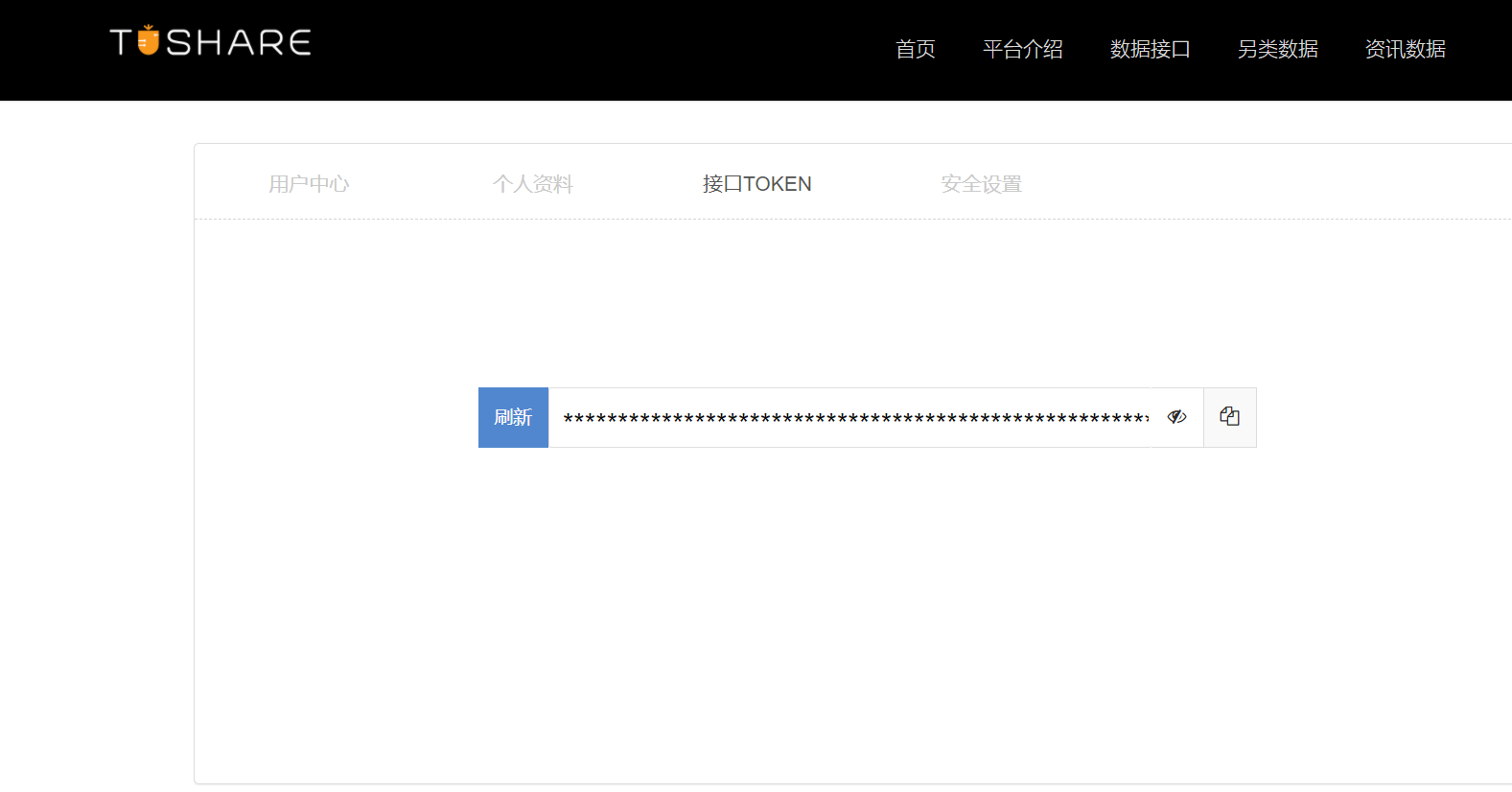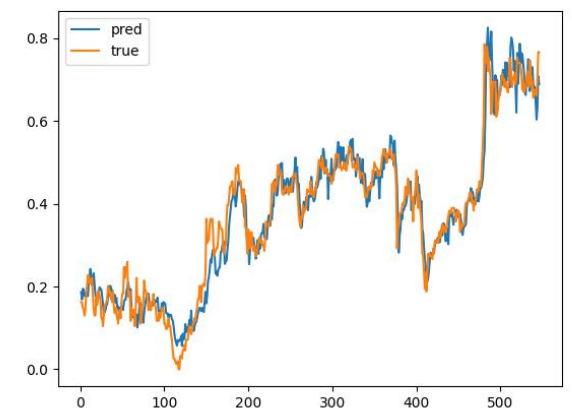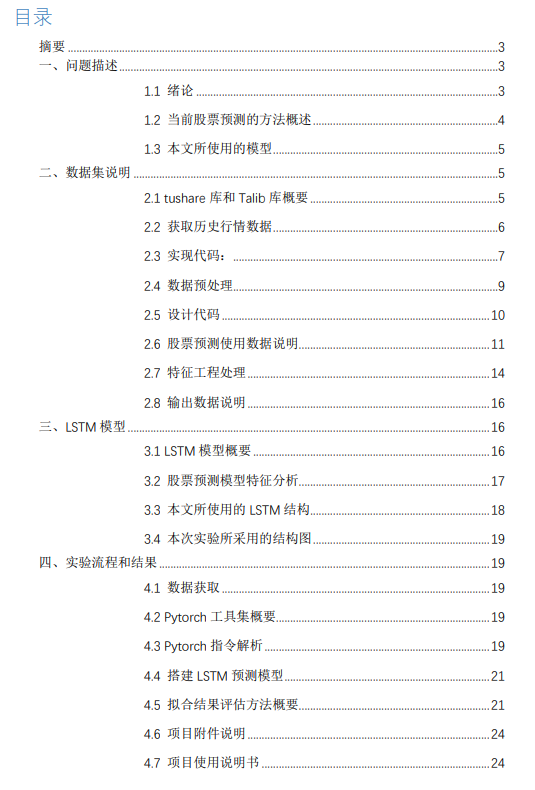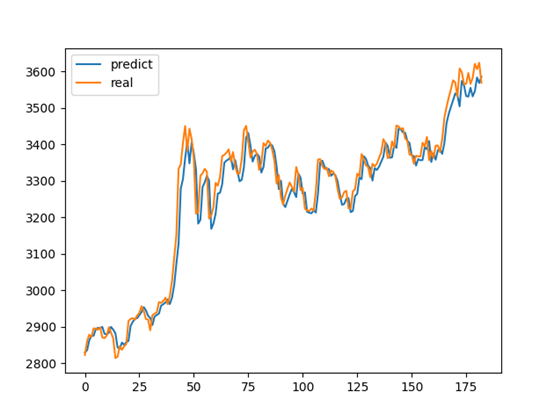• Tushare接口+LSTM模型预测股票走势 Tushare ID：423115 Tushare接口优势以及使用方法 Tushare是一款国内使用较为热门的财经接口，数据源稳定不易出错，速度较快，能符合开发的需求，下面讲讲使用的基本方法。 注册...
Tushare接口+LSTM模型预测股票走势
Tushare ID：423115
Tushare接口优势以及使用方法
Tushare是一款国内使用较为热门的财经接口，数据源稳定不易出错，速度较快，能符合开发的需求，下面讲讲使用的基本方法。

注册账号 通过注册账号可以获取属于个人的token码；python中import tushare 并且调用接口

import tushare as ts
ts.set_token('你的token码')
pro = ts.pro_api()

数据预处理
import numpy as np
from sklearn.preprocessing import MinMaxScaler
import tushare as ts
ts.set_token('你的token码')
pro = ts.pro_api()

#获取数据集
ts_code_temp = stock_config.STOCKCODE
df = pro.daily(ts_code = ts_code_temp, start_date='20160701')
df = df.sort_index(ascending=False)

df_close = df.reset_index()[['trade_date','close']]

#数据规则化
scaler=MinMaxScaler(feature_range=(0,1))
df_min=scaler.fit_transform(np.array(df_close['close']).reshape(-1,1))

#用于实际预测的数据集
df_lasttendays = df_min[-stock_config.timestep:]

LSTM模型
#LSTM
import data_tushare
import numpy as np
import matplotlib.pyplot as plt

T = 10 #学习天数
N = 3  #预测天数

df2 = data_tushare.df_min

##划分数据集
training_size=int(len(df2)*0.85)
test_size=len(df2)-training_size
train_data,test_data=df2[0:training_size,:],df2[training_size:len(df2),:1]

import numpy
# convert an array of values into a dataset matrix
def create_dataset(dataset, time_step=1):
dataX, dataY = [], []
for i in range(len(dataset)-time_step-1):
a = dataset[i:(i+time_step), 0]   ###i=0, 0,1,2,3-----9
dataX.append(a)
dataY.append(dataset[i + time_step, 0])
return numpy.array(dataX), numpy.array(dataY)

# reshape into X=t,t+1,t+2,t+3 and Y=t+4
time_step = T
X_train, y_train = create_dataset(train_data, time_step)
X_test, y_test = create_dataset(test_data, time_step)

# reshape input to be [samples, time steps, features] which is required for LSTM
X_train =X_train.reshape(X_train.shape,X_train.shape , 1)
X_test = X_test.reshape(X_test.shape,X_test.shape , 1)

### Create the Stacked LSTM model
from tensorflow.python.keras.models import Sequential
from tensorflow.python.keras.layers import Dense
from tensorflow.python.keras.layers import LSTM
model=Sequential()
model.add(LSTM(50,return_sequences=True,input_shape=(T,1)))
model.add(LSTM(50,return_sequences=True))
model.add(LSTM(50))
model.add(Dense(1))
model.compile(loss='mean_squared_error',optimizer='adam')

#开始拟合
model.fit(X_train,y_train,validation_data=(X_test,y_test),epochs=300,batch_size=45,verbose=1)

### Lets Do the prediction and check performance metrics
train_predict=model.predict(X_train)
test_predict=model.predict(X_test)

##Transformback to original form
train_predict = data_tushare.scaler.inverse_transform(train_predict)
test_predict = data_tushare.scaler.inverse_transform(test_predict)

### Calculate RMSE performance metrics
import math
from sklearn.metrics import mean_squared_error

### Test Data RMSE
rmse = math.sqrt(mean_squared_error(y_test,test_predict))
print(f'RMSE = {rmse}')

### Plotting

look_back=T
trainPredictPlot = numpy.empty_like(df2)
trainPredictPlot[:, :] = np.nan
trainPredictPlot[look_back:len(train_predict)+look_back, :] = train_predict
# shift test predictions for plotting
testPredictPlot = numpy.empty_like(df2)
testPredictPlot[:, :] = numpy.nan
testPredictPlot[len(train_predict)+(look_back*2)+1:len(df2)-1, :] = test_predict

plt.plot(data_tushare.scaler.inverse_transform(df2),'blue',label = "All data")
plt.plot(trainPredictPlot,'red',label = "Train data")
plt.plot(testPredictPlot,'green',label = "Test data")
plt.legend()
plt.show()

以上是数据清洗以及模型训练，之后的部分只要拉取合适的数据调用模型即可进行预测。


展开全文• 在我看来，该模型已经观察到了数据中的某些模式，因此它可以在大多数时候正确预测股票走势。但是，这个模型是否可以用于实际，有待用更多回测和实践去验证。 为什么需要时间序列模型? 你想要正确地模拟股票价格，...
前言
在本文开始前，作者并没有提倡LSTM是一种高度可靠的模型，它可以很好地利用股票数据中的内在模式，或者可以在没有任何人参与的情况下使用。写这篇文章，纯粹是出于对机器学习的热爱。在我看来，该模型已经观察到了数据中的某些模式，因此它可以在大多数时候正确预测股票的走势。但是，这个模型是否可以用于实际，有待用更多回测和实践去验证。

为什么需要时间序列模型?
你想要正确地模拟股票价格，因此作为股票买家，你可以合理地决定什么时候买股票，什么时候卖股票。这就是时间序列建模的切入点。你需要良好的机器学习模型，可以查看数据序列的历史记录，并正确地预测序列的未来元素是什么。

提示：股市价格高度不可预测且不稳定。这意味着在数据中没有一致的模式可以让你近乎完美地模拟股票价格。就像普林斯顿大学经济学家Burton Malkiel在他1973年的书中写到的：“随机漫步华尔街”，如果市场确实是有效的，那么当股票价格反应的所有因素一旦被公开时，那我们闭着眼睛都可以做的和专业投资者一样好。

但是，我们不要一直相信这只是一个随机过程，觉得机器学习是没有希望的。你不需要预测未来的股票确切的价格，而是股票价格的变动。做到这点就很不错了！

数据准备
使用以下数据源：
地址：https://www.kaggle.com/borismarjanovic/price-volume-data-for-all-us-stocks-etfs当然你也可基于Wind数据库去研究。因为Wind数据相对于其他平台和数据商而言，总体上在国内算是比较全面和准确的。

从Kaggle获得数据
在Kaggle上找到的数据是csv文件，所以，你不需要再进行任何的预处理，因此你可以直接将数据加载到DataFrame中。同时你还应该确保数据是按日期排序的，因为数据的顺序在时间序列建模中至关重要。
df = df.sort_values('Date')
df.head()数据可视化
plt.figure(figsize = (18,9))
plt.plot(range(df.shape),(df['Low']+df['High'])/2.0)
plt.xticks(range(0,df.shape,500),df['Date'].loc[::500],rotation=45)
plt.xlabel('Date',fontsize=18)
plt.ylabel('Mid Price',fontsize=18)
plt.show()上图已经说明了很多东西。我选择这家公司而不是其他公司的具体原因是，随着时间的推移，这张图中展现了不同的股价行为。这将使学习更加稳健，并且可以更改以便测试各种情况下预测的好坏程度。

数据拆分训练集和测试集
计算一天中最高和最低价的平均值来计算的中间价格。
high_prices = df.loc[:,'High'].as_matrix()
low_prices = df.loc[:,'Low'].as_matrix()
mid_prices = (high_prices+low_prices)/2.0


现在你可以分离训练数据和测试数据。训练数据是时间序列的前11000个数据，其余的是测试数据。
train_data = mid_prices[:11000]
test_data = mid_prices[11000:]


现在需要定义一个标准对数据进行归一化。MinMaxScalar方法将所有数据归到0和1之间。你还可以将训练和测试数据重新组为[data_size, num_features]。
scaler = MinMaxScaler()
train_data = train_data.reshape(-1,1)
test_data = test_data.reshape(-1,1)


根据之前得数据，可以看出不同时间段有不同的取值范围，你可以将整个序列拆分为窗口来进行归一化。如果不这样做，早期的数据接近于0，并且不会给学习过程增加太多价值。这里你选择的窗口大小是2500。

当选择窗口大小时，确保它不是太小，因为当执行窗口规范化时，它会在每个窗口的末尾引入一个中断，因为每个窗口都是**规范化的。

在本例中，4个数据点将受此影响。但假设你有11000个数据点，4个点不会引起任何问题。
smoothing_window_size = 2500
for di in range(0,10000,smoothing_window_size):
scaler.fit(train_data[di:di+smoothing_window_size,:])
train_data[di:di+smoothing_window_size,:] = scaler.transform(train_data[di:di+smoothing_window_size,:])

# You normalize the last bit of remaining data
scaler.fit(train_data[di+smoothing_window_size:,:])
train_data[di+smoothing_window_size:,:] = scaler.transform(train_data[di+smoothing_window_size:,:])


将数据重新塑造为[data_size]的Shape：
train_data = train_data.reshape(-1)
test_data = scaler.transform(test_data).reshape(-1)


现在可以使用指数移动平均平滑数据。可以帮助你避免股票价格数据的杂乱，并产生更平滑的曲线。我们只使用训练数据来训练MinMaxScaler，通过将MinMaxScaler与测试数据进行匹配来规范化测试数据是错误的。

注意：你应该只平滑训练数据。
EMA = 0.0
gamma = 0.1
for ti in range(11000):
EMA = gamma*train_data[ti] + (1-gamma)*EMA
train_data[ti] = EMA

all_mid_data = np.concatenate([train_data,test_data],axis=0)

下面是平均结果。它非常接近股票的实际行为。接下来您将看到一个更精确的一步预测方法：上面的图（和MSE）说明了什么呢？对于非常短的predictiosn（一天之后）来说，这个模型似乎不算太坏。考虑到股票价格在一夜之间不会从0变化到100，这种行为是明智的。接下来我们来看一种更有趣的平均技术，称为指数移动平均。

指数移动平均线
你可能在互联网上看到过一些文章使用非常复杂的模型来预测股票市场的行为。但是要小心！我所看到的这些都只是视觉错觉，不是因为学习了有用的东西。下面你将看到如何使用简单的平均方法复制这种行为。
window_size = 100
N = train_data.size

run_avg_predictions = []
run_avg_x = []

mse_errors = []

running_mean = 0.0
run_avg_predictions.append(running_mean)

decay = 0.5

for pred_idx in range(1,N):

running_mean = running_mean*decay + (1.0-decay)*train_data[pred_idx-1]
run_avg_predictions.append(running_mean)
mse_errors.append((run_avg_predictions[-1]-train_data[pred_idx])**2)
run_avg_x.append(date)

print('MSE error for EMA averaging: %.5f'%(0.5*np.mean(mse_errors)))

MSE error for EMA averaging: 0.00003如果指数移动平均线很好，为什么需要更好的模型呢？

可以看到，它符合遵循真实分布的完美直线（通过非常低的MSE证明了这一点）。实际上，仅凭第二天的股票市值，你就做不了什么。就我个人而言，我想要的不是第二天股市的确切价格，而是未来30天股市的价格会上涨还是下跌

让我们试着在窗口中进行预测（假设你预测接下来两天的窗口，而不是第二天）。然后你就会意识到EMA会有多么的失败。让我们通过一个例子来理解这一点。不管你预测未来的步骤是多少，你都会得到相同的答案。

输出有用信息的一种解决方案是查看基于动量算法。他们的预测是基于过去的近期值是上升还是下降（而不是精确的数值）。例如，如果过去几天的价格一直在下降，第二天的价格可能会更低。这听起来很合理。然而，我们将使用更复杂的模型：LSTM。

评价结果
我们将使用均值平方误差来计算我们的模型有多好。均值平方误差(MSE)的计算方法是先计算真实值与预测值之间的平方误差，然后对所有的预测进行平均。但是：

平均预测是一种很好的预测方法（这对股票市场的预测不是很有用），但对未来的预测并不是很有用。

LSTM简介
长短时记忆模型是非常强大的时间序列模型。它们可以预测未来任意数量的步骤。LSTM模块(或单元)有5个基本组件，可以对长期和短期数据进行建模。

LSTM单元格如下所示：计算方程如下：Tensorflow为实现时间序列模型提供了一个很好的子API。后面我们会使用到它。

LSTM数据生成器
首先要实现一个数据生成器来训练LSTM。这个数据生成器将有一个名为unroll_batch(…)的方法，该方法将输出一组按顺序批量获取num_unrollings的输入数据，其中批数据的大小为[batch_size, 1]。然后每批输入数据都有相应的输出数据。

例如，如果num_unrollings=3和batch_size=4则看起来像一组展开的批次。
输入数据： [x0,x10,x20,x30],[x1,x11,x21,x31],[x2,x12,x22,x32]
输出数据： [x1,x11,x21,x31],[x2,x12,x22,x32],[x3,x13,x23,x33]

数据生成器
下面将演示如何可视化创建一批数据。基本思想是将数据序列划分为N / b段，使每个段的大小为b，然后定义游标每段为1。然后对单个数据进行抽样，我们得到一个输入（当前段游标索引）和一个真实预测（在[当前段游标+1，当前段游标+5]之间随机抽样）。请注意，我们并不总是得到输入旁边的值，就像它的预测一样。这是一个减少过拟合的步骤。在每次抽样结束时，我们将光标增加1。定义超参数
在本节中，将定义几个超参数。D是输入的维数。很简单，你以之前的股票价格作为输入并预测下一个应该为1。然后是num_unrollings，它表示单个优化步骤需要考虑多少连续时间步骤。越大越好。然后是batch_size。批量处理大小是在单个时间步骤中考虑的数据样本的数量。越大越好，因为在给定的时间内数据的可见性越好。接下来定义num_nodes，它表示每个单元格中隐藏的神经元数量。在这个示例中，你可以看到有三层LSTM。
D = 1
num_unrollings = 50
batch_size = 500
num_nodes = [200,200,150]
n_layers = len(num_nodes)
dropout = 0.2

tf.reset_default_graph()


定义输入和输出
接下来为训练输入和标签定义占位符。这非常简单，因为你有一个输入占位符列表，其中每个占位符包含一批数据。 该列表包含num_unrollings占位符，它将用于单个优化步骤。
train_inputs, train_outputs = [],[]
for ui in range(num_unrollings):
train_inputs.append(tf.placeholder(tf.float32, shape=[batch_size,D],name='train_inputs_%d'%ui))
train_outputs.append(tf.placeholder(tf.float32, shape=[batch_size,1], name = 'train_outputs_%d'%ui))


定义LSTM和回归层的参数
用三个LSTM层和一个线性回归层，用w和b表示，该层提取最后一个长短期内存单元的输出并输出对下一个时间步骤的预测。你可以使用TensorFlow中的MultiRNNCell来封装创建的三个LSTMCell对象。此外，还可以使用dropout实现LSTM单元格，因为它们可以提高性能并减少过拟合。
lstm_cells = [
tf.contrib.rnn.LSTMCell(num_units=num_nodes[li],
state_is_tuple=True,
initializer= tf.contrib.layers.xavier_initializer()
)
for li in range(n_layers)]

drop_lstm_cells = [tf.contrib.rnn.DropoutWrapper(
lstm, input_keep_prob=1.0,output_keep_prob=1.0-dropout, state_keep_prob=1.0-dropout
) for lstm in lstm_cells]
drop_multi_cell = tf.contrib.rnn.MultiRNNCell(drop_lstm_cells)
multi_cell = tf.contrib.rnn.MultiRNNCell(lstm_cells)

w = tf.get_variable('w',shape=[num_nodes[-1], 1], initializer=tf.contrib.layers.xavier_initializer())
b = tf.get_variable('b',initializer=tf.random_uniform(,-0.1,0.1))


计算LSTM输出并将其输入回归层，得到最终预测结果
首先创建TensorFlow变量（c和h），它将保持单元状态和长短期记忆单元的隐藏状态。 然后将train_input列表转换为[num_unrollings, batch_size, D]，使用tf.nn.dynamic_rnn计算所需输出。然后使用tf.nn.dynamic_rnn计算LSTM输出。并将输出分解为一列num_unrolling的张量。预测和真实股价之间的损失。
c, h = [],[]
initial_state = []
for li in range(n_layers):
c.append(tf.Variable(tf.zeros([batch_size, num_nodes[li]]), trainable=False))
h.append(tf.Variable(tf.zeros([batch_size, num_nodes[li]]), trainable=False))
initial_state.append(tf.contrib.rnn.LSTMStateTuple(c[li], h[li]))

all_inputs = tf.concat([tf.expand_dims(t,0) for t in train_inputs],axis=0)

all_lstm_outputs, state = tf.nn.dynamic_rnn(
drop_multi_cell, all_inputs, initial_state=tuple(initial_state),
time_major = True, dtype=tf.float32)

all_lstm_outputs = tf.reshape(all_lstm_outputs, [batch_size*num_unrollings,num_nodes[-1]])

all_outputs = tf.nn.xw_plus_b(all_lstm_outputs,w,b)

split_outputs = tf.split(all_outputs,num_unrollings,axis=0)


损失计算和优化器
现在，要计算损失。然而，在计算损失时，你应该注意到有一个独特的特征。对于每一批预测和真实输出，计算均方误差。然后把所有这些均方损失加起来（不是平均值）。最后，定义要用来优化神经网络的优化器在这种情况下，您可以使用Adam，这是一个非常新且性能良好的优化器。
print('Defining training Loss')
loss = 0.0
with tf.control_dependencies([tf.assign(c[li], state[li]) for li in range(n_layers)]+
[tf.assign(h[li], state[li]) for li in range(n_layers)]):
for ui in range(num_unrollings):
loss += tf.reduce_mean(0.5*(split_outputs[ui]-train_outputs[ui])**2)

print('Learning rate decay operations')
global_step = tf.Variable(0, trainable=False)
inc_gstep = tf.assign(global_step,global_step + 1)
tf_learning_rate = tf.placeholder(shape=None,dtype=tf.float32)
tf_min_learning_rate = tf.placeholder(shape=None,dtype=tf.float32)

learning_rate = tf.maximum(
tf.train.exponential_decay(tf_learning_rate, global_step, decay_steps=1, decay_rate=0.5, staircase=True),
tf_min_learning_rate)

# Optimizer.
print('TF Optimization operations')
optimizer = tf.train.AdamOptimizer(learning_rate)
gradients, v = zip(*optimizer.compute_gradients(loss))
gradients, _ = tf.clip_by_global_norm(gradients, 5.0)
optimizer = optimizer.apply_gradients(
zip(gradients, v))

print('\tAll done')

这里定义了与预测相关的TensorFlow操作。首先，为输入（sample_inputs）定义一个占位符，然后与训练阶段类似，定义预测的状态变量（sample_c和sample_h）。最后用tf.nn.dynamic_rnn计算预测。后通过回归层（w和b）发送输出。 还应该定义reset_sample_state操作，该操作将重置单元状态和隐藏状态。 每次进行一系列预测时，都应该在开始时执行此操作。

print('Defining prediction related TF functions')

sample_inputs = tf.placeholder(tf.float32, shape=[1,D])

sample_c, sample_h, initial_sample_state = [],[],[]
for li in range(n_layers):
sample_c.append(tf.Variable(tf.zeros([1, num_nodes[li]]), trainable=False))
sample_h.append(tf.Variable(tf.zeros([1, num_nodes[li]]), trainable=False))
initial_sample_state.append(tf.contrib.rnn.LSTMStateTuple(sample_c[li],sample_h[li]))

reset_sample_states = tf.group(*[tf.assign(sample_c[li],tf.zeros([1, num_nodes[li]])) for li in range(n_layers)],
*[tf.assign(sample_h[li],tf.zeros([1, num_nodes[li]])) for li in range(n_layers)])

sample_outputs, sample_state = tf.nn.dynamic_rnn(multi_cell, tf.expand_dims(sample_inputs,0),
initial_state=tuple(initial_sample_state),
time_major = True,
dtype=tf.float32)

with tf.control_dependencies([tf.assign(sample_c[li],sample_state[li]) for li in range(n_layers)]+
[tf.assign(sample_h[li],sample_state[li]) for li in range(n_layers)]):
sample_prediction = tf.nn.xw_plus_b(tf.reshape(sample_outputs,[1,-1]), w, b)

print('\tAll done')


运行LSTM
在这里，你将训练和预测几个时期的股票价格走势，看看这些预测是否会随着时间的推移而变得更好或更糟。按照以下步骤操作：

在时间序列上定义一组测试起点（test_points_seq）来计算LSTM
对于每一个epoch

用于训练数据的完整序列长度

展开一组num_unrollings批次
使用展开的批次LSTM进行训练

计算平均训练损失
对于测试集中的每个起点

通过迭代在测试点之前找到的以前的num_unrollings数据点来更新LSTM状态
使用先前的预测作为当前输入，连续预测n_predict_once步骤
计算预测到的n_predict_once点与当时股票价格之间的MSE损失

部分代码
epochs = 30
valid_summary = 1
n_predict_once = 50
train_seq_length = train_data.size
train_mse_ot = []
test_mse_ot = []
predictions_over_time = []
session = tf.InteractiveSession()
tf.global_variables_initializer().run()
loss_nondecrease_count = 0
loss_nondecrease_threshold = 2

print('Initialized')
average_loss = 0
data_gen = DataGeneratorSeq(train_data,batch_size,num_unrollings)
x_axis_seq = []
test_points_seq = np.arange(11000,12000,50).tolist()

for ep in range(epochs):

# ========================= Training =====================================
for step in range(train_seq_length//batch_size):

u_data, u_labels = data_gen.unroll_batches()

feed_dict = {}
for ui,(dat,lbl) in enumerate(zip(u_data,u_labels)):
feed_dict[train_inputs[ui]] = dat.reshape(-1,1)
feed_dict[train_outputs[ui]] = lbl.reshape(-1,1)

feed_dict.update({tf_learning_rate: 0.0001, tf_min_learning_rate:0.000001})

_, l = session.run([optimizer, loss], feed_dict=feed_dict)


可视化预测
可以看到MSE损失是如何随着训练量的减少而减少的。这是一个好迹象，表明模型正在学习一些有用的东西。你可以看到LSTM比标准平均值做得更好。标准平均（虽然不完美）合理地跟随真实的股票价格运动。
best_prediction_epoch = 28
plt.figure(figsize = (18,18))
plt.subplot(2,1,1)
plt.plot(range(df.shape),all_mid_data,color='b')

predictions with high alpha
start_alpha = 0.25
alpha  = np.arange(start_alpha,1.1,(1.0-start_alpha)/len(predictions_over_time[::3]))
for p_i,p in enumerate(predictions_over_time[::3]):
for xval,yval in zip(x_axis_seq,p):
plt.plot(xval,yval,color='r',alpha=alpha[p_i])

plt.title('Evolution of Test Predictions Over Time',fontsize=18)
plt.xlabel('Date',fontsize=18)
plt.ylabel('Mid Price',fontsize=18)
plt.xlim(11000,12500)

plt.subplot(2,1,2)

plt.plot(range(df.shape),all_mid_data,color='b')
for xval,yval in zip(x_axis_seq,predictions_over_time[best_prediction_epoch]):
plt.plot(xval,yval,color='r')

plt.title('Best Test Predictions Over Time',fontsize=18)
plt.xlabel('Date',fontsize=18)
plt.ylabel('Mid Price',fontsize=18)
plt.xlim(11000,12500)
plt.show()尽管LSTM并不完美，但它似乎在大多数情况下都能正确预测股价走势。请注意，你的预测大致在0和1之间（也就是说，不是真实的股票价格）。这是可以的，因为你预测的是股价的走势，而不是股价本身。

结论
股票价格/移动预测是一项极其困难的任务。就我个人而言，我认为任何股票预测模型都不应该被视为理所当然，并且盲目地依赖它们。然而，模型在大多数情况下可能能够正确预测股票价格的变动，但并不总是如此。

不要被那些预测曲线完全与真实股价重叠的文章所迷惑。这可以用一个简单的平均技术来复制，但实际上它是无用的。更明智的做法是预测股价走势。

模型的超参数对你得到的结果非常敏感。因此，一个非常好的事情是在超参数上运行一些超参数优化技术（例如，网格搜索/随机搜索）。这里列出了一些最关键的超参数：优化器的学习率、层数、每层的隐藏单元数，优化器Adam表现最佳，模型的类型（GRU / LSTM / LSTM with peepholes）。

由于本文由于数据量小，我们用测试损耗来衰减学习速率。这间接地将测试集的信息泄露到训练过程中。处理这个问题更好的方法是有一个单独的验证集（除了测试集）与验证集性能相关的衰减学习率。

延伸阅读：
使用Tensorflow预测股票市场变动


展开全文Tensorflow
• 下图是基于LSTM的对股票走势预测的结果 项目附件说明 本次实验项目文件有以下文件夹：stockPredict 文件夹。 1、根目录下存放使用的 python 代码。 2、data 文件夹下存放获取到的股票数据。 3、model 文件夹中...
下图是基于LSTM的对股票走势的预测的结果项目附件说明
本次实验项目文件有以下文件夹：stockPredict 文件夹。
1、根目录下存放使用的 python 代码。
2、data 文件夹下存放获取到的股票数据。
3、model 文件夹中包含已经训练好的模型。
4、img 文件夹中包含测试数据表现折线图和实验结构图。
项目使用说明书
本次实验项目使用方法对用户十分友好，只需要作如下操作：
1、在 parser_my 中修改需要的参数，如数据源文件，LSTM 参数等。
2、train 文件用于训练。模型会自动保存。
3、test 文件用于测试，结果会自动保存。
4、draw 文件用于获取准确率和画出走势图，提供使用者比对
基于LSTM的对股票走势的预测项目有问题可以callme
基于LSTM的对股票走势的预测项目有问题可以callme
基于LSTM的对股票走势的预测项目有问题可以callme
基于LSTM的对股票走势的预测项目有问题可以callme
基于LSTM的对股票走势的预测项目有问题可以callme
基于LSTM的对股票走势的预测项目有问题可以callme
链接：https://pan.baidu.com/s/1eNxDJMPGqXqNEuwgCZXN2Q
提取码：jyz0
复制这段内容后打开百度网盘手机App，操作更方便哦


展开全文python 机器学习 tensorflow java 深度学习
• 现阶段国内股票市场散户市和政策市特征明显，市面上没有一种准确有效输出直观的工具来帮助散户进行股票价格预测，且大部分预测模型还是以精度不高的传统计量经济学模型或者BP神经网络为基础。本项目拟结合数据面和...
项目简介与开发背景
现阶段国内股票市场散户市和政策市特征明显，市面上没有一种准确有效输出直观的工具来帮助散户进行股票价格预测，且大部分预测模型还是以精度不高的传统计量经济学模型或者BP神经网络为基础。本项目拟结合数据面和政策面的影响，以长短期记忆网络为基础，以提高精度并克服BP神经网络的缺点，同时通过语义分析技术将传统模型所没有的国家政策、股民情绪、媒体评判等量化后作为输入的一部分。此外将国际金融市场走势，国际时局等作为输入的一部分，并通过对所有输入的数据挖掘来简化输入，最后引入交易模型，直观输出股指以帮助股民规避风险做出判断。
tushare ID：419950
数据获取与处理
tushare介绍
tushare提供了基于python的金融数据接口，是一个免费、开放的数据平台。数据内容包括股票、基金、期货、债券、外汇、行业大数据，同时包括了数字货币行情等区块链数据的全数据品类的金融大数据平台，为各类金融投资和研究人员提供适用的数据和工具。
同时，也为高校学生提供了便利的服务，所以在进行这次项目时数据来源就选择了tushare，也十分推荐大家使用。
数据获取
本项目对上证指数(000001.SH)进行预测，获取了仅二十余年的数据，数据来源于开源社区tushare
代码如下
def dataget(start_date, end_date, code):
"""
数据获取
:param start_date: string
:param end_date: string
:param code: string
:return: ndarray
"""
ts.set_token("your token")
pro = ts.pro_api()
df = pro.index_daily(ts_code=code, start_date=start_date, end_date=end_date,
fields='trade_date,close,open,high,low,vol,amount,pct_chg')
length = df.shape
data = []
for i in range(length):
each_data = [df.loc[length - 1 - i]['trade_date'], df.loc[length - 1 - i]['open'], df.loc[length - 1 - i]['close'], df.loc[length - 1 - i]['low'],
df.loc[length - 1 - i]['high'], df.loc[length - 1 - i]['vol'], df.loc[length - 1 - i]['amount'],
df.loc[length - 1 - i]['pct_chg']]
each_data = np.array(each_data, dtype='float64')
data.append(each_data)
data = np.array(data, dtype='float64')
print('数据类型：', type(data))
print('数据个数：', data.shape)
print('数据形状：', data.shape)
print('数据第一行：', data)
return data

本项目获取的数据有日期、开盘价、收盘价、最高价、最低价等。
数据处理
数据处理主要依赖于归一化处理。数据的标准化（normalization）是将数据按比例缩放，使之落入一个小的特定区间。在某些比较和评价的指标处理中经常会用到，去除数据的单位限制，将其转化为无量纲的纯数值，便于不同单位或量级的指标能够进行比较和加权。
归一化公式:
x*=(x-avg)/(max-min)
模型搭建
在搭建模型时花费了较大的功夫进行评估，最后评估结果为一层LSTM+2层全连接会比较好，模型如下
    model = Sequential()
# LSTM层
model.add(LSTM(256, activation='relu', return_sequences=False, input_shape=(layers, layers),
kernel_regularizer=regularizers.l2(0.000003)))
model.add(Dropout(0.3))
# BP层
model.add(Dense(128))
model.add(Activation("relu"))
model.add(Dropout(0.5))
# 输出层
model.add(Dense(layers))
model.add(Activation("linear"))
# 优化器
op = tensorflow.keras.optimizers.Adam()
# 损失函数
lo = tensorflow.keras.losses.MeanSquaredLogarithmicError()
model.compile(optimizer=op, loss=lo)

预测效果
验证集与预测结果的对比如下后记
本文展示的是基于日线指数的股票预测，笔者还进行了加上情感指数的股票预测，主要思路是爬取每天新闻，对新闻进行情感指数分类，输入到神经网络中进行股票预测。新闻的获取也是来源于tushare，tushare对于这一类的接口还是很齐全的。
完整代码可见GitHub:https://github.com/foragony/stock_predict


展开全文神经网络 python 深度学习
• 本来做的美股预测，但是没有本地特色，于是乎改用中国，所以在接口上有所改变，故使用的时候，改变接口就可以完美配合所有股市数据。 # Import import tensorflow as tf import numpy as np import pandas as pd ...大数据 tensorflow 机器学习 python
• LSTM数据集实战 ...既然是时间序列预测，我们最关心的是预测值在时间维度上的走势如何，那我们只要最后一列volume和第一列date这两列就好了。 实战 先是导入相关包，一些常见的包就不详细说了，我们需要的Sepython 深度学习 人工智能
• 论文代码“通过对抗训练增强股票走势预测” IJCAI 2019 要求 的Python 3.6.1 Tensorflow 1.8.0 脾气暴躁的1.14.5 要在ACL18和KDD17数据集上运行建议的Adv-ALSTM，ALSTMLSTM，请运行超参数文件中的命令。 参考 ...
• 首先通过DMD算法对受市场板块联动效应影响的关联行业板块样本股数据进行分解计算，提取包含整体市场和特定股票走势变化信息的模态特征；然后针对不同市场背景，采用LSTM网络对基本面数据和模态特征进行价格建模预测...
• 最近研究了一下用深度学习算法来预测股票未来的走势，看了网上不少别人分享的案例，也实际进行了测试，感觉用 LSTM 算法比较适用。长短期记忆网络（LSTM，Long Short-Term Memory）是一种时间循环神经网络，是为了...机器学习 深度学习
• 事实上，金融专家对股票价值预测的检查领域非常感兴趣。 对于体面而有用的投机，众多投机者对股市未来走势了如指掌。 强大而强大的股票市场预测框架可帮助交易商、投机者和专家提供重要数据，例如股票市场的未来...
• 本文主要对LSTM模型结构改进及优化其参数, 使其预测股票涨跌走势准确率明显提高, 同时对美股周数据及日数据在LSTM神经网络预测效果展开研究. 一方面通过分析对比两者预测效果差别, 验证不同数据集对预测效果的影响; ...
• 文章来源：bigquan摘要：BigQuant3平台上的StockRanker算法在选股方面有不俗的表现，模型在15、16年的回测收益率也很高(使用默认因子收益率就... LSTM(长短期记忆神经网络)是一种善于处理和预测时间序列相关数据的RN...量化交易 交易策略
• 潜在的未来股价走势，并因此对要分析的股票做出买/卖/持有决定。 这里包括的工具不是唯一可以使用的工具。 之所以将它们包括在内，是因为我相信没有任何一种工具或模型可以充分理解导致股价波动的所有因素。 此...
• 摘要：BigQuant人工智能量化投资平台上的StockRanker算法在选股方面有不俗的表现，模型在15、16年的回测收益率也很高(使用默认因子收益... LSTM(长短期记忆神经网络)是一种善于处理和预测时间序列相关数据的RNN。本...人工智能 量化投资
• 前言 疫情期间，我爸妈又开始炒股了，鉴于之前做过一个AI结合的量化交易项目，但是不是负责算法部分，所以想...明白预测股市走势的动机； 下载股票数据 — 你将使用由 Alpha Vantage 或 Kaggle 收集的股票数据； 将数.自然语言处理 神经网络 数据挖掘 机器学习 深度学习
• 预测未来股价走势是一个再好不过的例子了。在本文中，我们将看到如何在递归神经网络的帮助下执行时间序列分析。我们将根据过去5年的股价预测苹果公司之后的股价。 数据集 本文的数据可以从雅虎财经下载。我们将...
• AI科技评论按：BigQuant平台上的 StockRanker 算法在选股方面有不俗的表现，模型在 15、16 年的回测收益率也很高 (使用默认因子收益率就达到 170% ... LSTM(长短期记忆神经网络) 是一种善于处理和预测时间序列相关数神经网络
• 摘要： 序列预测问题曾经被认为是数据科学行业最难解决的问题之一，而LSTM则是解决这类问题的必备法宝。...涉及序列问题的包括：预测销售、发现股票市场走势、了解电影情节、了解你的演讲方式、语言翻译、
• 全文共4109字，预计学习时长8分钟 从在线API中提取股票价格数据，使用配备有...该实验使用人工神经网络揭示股市走势，揭示了使用过去的历史数据来预测未来股票价格的能力，即时间序列预测能力。 注意！！...人工智能 代码
• 经过研究人员和金融专家对股票市场预测的多年研究之后，似乎没有确定的方法可以既准确又持续地预测股票价格。 这是由于股票走势的不确定性以及决定市场表现的众多参数所致。 人们已经研究了许多技术来预测股市价格。...
• 大家可以根据预测股票走势做参考，来进行投资。也可以基于我的模型基础上进一步完善和优化，所以价值是非常高的。本课程由浅到深讲解，分为简单股票模型和复杂股票模型，模型的效果也是非常好，最终的效果如下：1...机器学习 机器学习算法 机器学习实战 python机器学习
• 论文核心思想：本篇论文主要比较了Back propogation,SVM,LSTM预测股票趋势时的效果，最后得出结论LSTM在预测走势时的结果较好，，，，这个paper太水了。。 转载于:https://www.cnblogs.com/...
• 我们部署了 CNN 网络来预测 1994 年至 2014 年间 200 只大宗欧洲股票的样本外股票走势，并将其整体表现与 Fischer, Krauss (2017) 中修改的 LSTM 模型进行比较。 具体来说，我们比较了两种模型架构的经验训练和验证...
• #基于LSTM模型预测股票价格走势 导入聚宽函数库 import jqdata 初始化函数，设定要操作的股票、基准等等 def initialize(context): # 通过模型预测2017-05-13 到 2018-03-24的收盘价格 context.labels = [3484.4731,...预测股价 股价预测 机器学习量化策略
• 我们的目标是使用组织的报告提供的数据来预测股票至少2个月的价格走势。 项目目标： 准确预测股票的未来价格走势。 通过网络实施程序 改进该程序可以产生一个人工智能交易机器人来主导对冲基金行业。 小组成员 ...